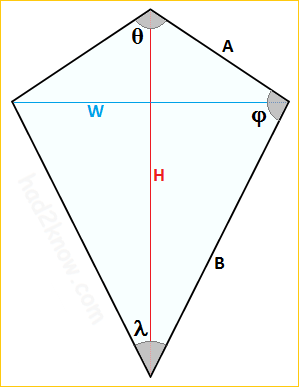# How to Compute the Area of a Kite

 Kite Area Calculator Fill in either WH, ABθ, ABφ, or ABλ W =   H =   A =   B =   θ = ° φ = ° λ = °A kite is a quadrilateral with two pairs of sides that have equal length. In a kite, the sides of equal length are adjacent to one another. (If equal sides are opposite to one another, the figure is a parallelogram.)

There are several formulas for computing the area of a kite depending on which measurements are known. Each formula is explained below and references the diagram below the calculator on the left.

Equation 1:
Height and Width

The width of a kite is the shorter distance between opposite points and the height is the greater distance between the other pair of opposite points. If we represent the two measurements by W and H respectively, then the area of the kite is (1/2)WH.

To see why this is so, imagine drawing a rectangle around the kite with the longer side parallel to the kite's height, the shorter side parallel to the kite's width, and the points of the kite on the rectangle's perimeter.

The kite takes up exactly 1/2 of the area of the rectangle. The triangular regions inside the rectangle and outside of the kite can be rearranged to form another kite of equal size and shape.

Equation 2:
Sides Lengths and Side Angle

Suppose the two shorter sides of the kite have length A and the two longer sides have length B, and call the angle between two unequal sides φ. (See diagram.)

Using the SAS formula for the area of a triangle, we can see that half of the kite has an area of (1/2)ABsin(φ). Since there are two halves, the total area is ABsin(φ).

Equation 3:
Sides Lengths and Top/Bottom Angle

Suppose you know the side lengths of the kite and one of either the top or bottom angles. For the sake of example, let's say the known angle is θ which is the angle formed by two shorter sides with length A.

Using trigonometry, we can deduce that the total width of the kite is 2Asin(θ/2). The partial height of the kite is Acos(θ/2).

To find the rest of the height, we use the Pythagorean theorem with B as the hypotenuse and Asin(θ/2) as one of the legs. This gives the rest of the height as sqrt(B² - A²sin²(θ/2)).

Thus, the total height is Acos(θ/2) + sqrt(B² - A²sin²(θ/2)).

This means the total area is

(1/2) * 2Asin(θ/2) * [Acos(θ/2) + sqrt(B² - A²sin²(θ/2))]

= Asin(θ/2)[Acos(θ/2) + sqrt(B² - A²sin²(θ/2))]

By the same argument, if you know A, B, and λ, the area is

Bsin(λ/2)[Bcos(λ/2) + sqrt(A² - B²sin²(λ/2))]